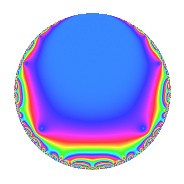# Properties

 Label 99.2.a.dLevel 99 Weight 2 Character orbit 99.a Self dual Yes Analytic conductor 0.791 Analytic rank 0 Dimension 1 CM No Inner twists 1

# Related objects

## Newspace parameters

 Level: $$N$$ = $$99 = 3^{2} \cdot 11$$ Weight: $$k$$ = $$2$$ Character orbit: $$[\chi]$$ = 99.a (trivial)

## Newform invariants

 Self dual: Yes Analytic conductor: $$0.790518980011$$ Analytic rank: $$0$$ Dimension: $$1$$ Coefficient field: $$\mathbb{Q}$$ Coefficient ring: $$\mathbb{Z}$$ Coefficient ring index: $$1$$ Fricke sign: $$-1$$ Sato-Tate group: $\mathrm{SU}(2)$

## $q$-expansion

 $$f(q)$$ $$=$$ $$q + 2q^{2} + 2q^{4} - q^{5} - 2q^{7} + O(q^{10})$$ $$q + 2q^{2} + 2q^{4} - q^{5} - 2q^{7} - 2q^{10} - q^{11} + 4q^{13} - 4q^{14} - 4q^{16} + 2q^{17} - 2q^{20} - 2q^{22} + q^{23} - 4q^{25} + 8q^{26} - 4q^{28} + 7q^{31} - 8q^{32} + 4q^{34} + 2q^{35} + 3q^{37} + 8q^{41} - 6q^{43} - 2q^{44} + 2q^{46} - 8q^{47} - 3q^{49} - 8q^{50} + 8q^{52} + 6q^{53} + q^{55} - 5q^{59} + 12q^{61} + 14q^{62} - 8q^{64} - 4q^{65} - 7q^{67} + 4q^{68} + 4q^{70} + 3q^{71} + 4q^{73} + 6q^{74} + 2q^{77} - 10q^{79} + 4q^{80} + 16q^{82} + 6q^{83} - 2q^{85} - 12q^{86} - 15q^{89} - 8q^{91} + 2q^{92} - 16q^{94} - 7q^{97} - 6q^{98} + O(q^{100})$$

## Embeddings

For each embedding $$\iota_m$$ of the coefficient field, the values $$\iota_m(a_n)$$ are shown below.

For more information on an embedded modular form you can click on its label.

Label $$\iota_m(\nu)$$ $$a_{2}$$ $$a_{3}$$ $$a_{4}$$ $$a_{5}$$ $$a_{6}$$ $$a_{7}$$ $$a_{8}$$ $$a_{9}$$ $$a_{10}$$
1.1
 0
2.00000 0 2.00000 −1.00000 0 −2.00000 0 0 −2.00000
 $$n$$: e.g. 2-40 or 990-1000 Significant digits: Format: Complex embeddings Normalized embeddings Satake parameters Satake angles

## Inner twists

This newform does not admit any (nontrivial) inner twists.

## Atkin-Lehner signs

$$p$$ Sign
$$3$$ $$-1$$
$$11$$ $$1$$

## Hecke kernels

This newform can be constructed as the intersection of the kernels of the following linear operators acting on $$S_{2}^{\mathrm{new}}(\Gamma_0(99))$$:

 $$T_{2} - 2$$ $$T_{5} + 1$$